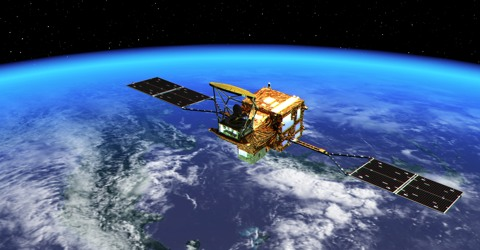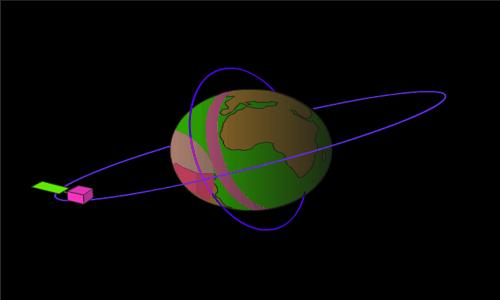Physics

# Principle of Artificial Satellites operationThe principle of Artificial Satellites operation

The satellite that is created by man based on this theory in order to go in the outer space is called artificial satellite. It is a man-made device placed in orbit around the earth, moon, or another planet used for communication and transmitting scientific information to earth.

In order to place the artificial satellite in the orbit with the necessary speed, the rocket is used. To avoid air resistance with the help of the rocket the satellite is placed in an orbit a few hundred kilometers above the earth’s surface. As the density of air is maximum on earth’s surface, so resistance on the motion of the rocket is also maximum. For traveling shortest distance of this part, the rocket first ascends vertically upward and then by remote control the rocket is made to incline slowly. This control is done in such a way that after reaching the desired orbit the rocket provides necessary revolving speed to the satellite. This time the satellite is separated from the rocket by remote control. Then the satellite starts revolving around the earth. In the figure, different orbit for rotating satellites has been shown.Fig: Orbits of rotating the artificial satellite

Artificial satellites revolve around the earth in such a way as its centrifugal or outward force gives external motion, but gravitational force of the earth does not allow it to go outside. Both the forces establish balance in the motion of artificial satellite and the artificial satellite revolves around the earth. Since there is no existence of air in the space so it can move without friction as long as it does not enter into the nearest place of the earth and upper region atmosphere pulls the artificial satellite downward and makes its motion slow. Artificial satellites do not travel in circular orbits; rather they travel in elliptical orbits. Satellites used for transmitting TV and Radio signals and weather observatory satellites normally stay about 36 thousands kilometers distance away from the earth. The velocity of an artificial satellite does not depend on its mass, rather the velocity increases as the distance from the surface of the earth to the satellite increases.

That means, v ∞ 1/√r

Velocity and time period of an artificial satellite can be determined from the following equation:

v = √(GM/r) = √[GM/(R+h)]

and, T = 2π(R+h) √[(R+h)/GM]

Here, R = radius of the earth, M = mass of the earth, G = gravitational constant.